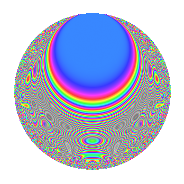# Properties

 Label 403.2.s.aLevel 403 Weight 2 Character orbit 403.s Analytic conductor 3.218 Analytic rank 0 Dimension 70 CM No

# Related objects

## Newspace parameters

 Level: $$N$$ = $$403 = 13 \cdot 31$$ Weight: $$k$$ = $$2$$ Character orbit: $$[\chi]$$ = 403.s (of order $$6$$ and degree $$2$$)

## Newform invariants

 Self dual: No Analytic conductor: $$3.21797120146$$ Analytic rank: $$0$$ Dimension: $$70$$ Relative dimension: $$35$$ over $$\Q(\zeta_{6})$$ Sato-Tate group: $\mathrm{SU}(2)[C_{6}]$

## $q$-expansion

The dimension is sufficiently large that we do not compute an algebraic $$q$$-expansion, but we have computed the trace expansion.

 $$\operatorname{Tr}(f)(q) =$$ $$70q - 6q^{2} - 2q^{3} + 30q^{4} - 29q^{9} + O(q^{10})$$ $$\operatorname{Tr}(f)(q) =$$ $$70q - 6q^{2} - 2q^{3} + 30q^{4} - 29q^{9} + 2q^{10} + 13q^{12} + q^{13} - 14q^{14} - 15q^{15} - 28q^{16} - 12q^{17} - 3q^{20} - 9q^{21} + 4q^{22} + 10q^{23} + 18q^{24} + 19q^{25} + 6q^{26} + 34q^{27} - 33q^{28} - 18q^{29} - 31q^{30} - 2q^{31} + 36q^{32} - 12q^{33} + 9q^{34} - 12q^{35} - 16q^{36} - 18q^{37} - 21q^{38} - 30q^{39} + 5q^{40} + 98q^{42} - 38q^{43} + 42q^{44} - 6q^{46} + 54q^{48} - 18q^{49} - 51q^{50} - 7q^{51} + 41q^{52} - 22q^{53} + 18q^{54} - 15q^{55} - 50q^{56} + 15q^{57} - 12q^{58} - 13q^{61} - 23q^{62} - 6q^{63} - 38q^{64} - 12q^{65} - 52q^{66} - 44q^{68} + 32q^{69} + 27q^{71} - 15q^{72} - 9q^{73} + 38q^{74} - 50q^{75} + 126q^{76} + 34q^{77} + 14q^{78} + 6q^{79} - 11q^{81} + 39q^{82} - 54q^{83} + 15q^{84} - 33q^{85} - 24q^{86} + 28q^{87} - 32q^{88} - 6q^{89} - 11q^{90} - 70q^{91} - 6q^{92} + 14q^{93} - 43q^{94} + 25q^{95} + 36q^{96} - 75q^{97} + 93q^{99} + O(q^{100})$$

## Embeddings

For each embedding $$\iota_m$$ of the coefficient field, the values $$\iota_m(a_n)$$ are shown below.

For more information on an embedded modular form you can click on its label.

Label $$a_{2}$$ $$a_{3}$$ $$a_{4}$$ $$a_{5}$$ $$a_{6}$$ $$a_{7}$$ $$a_{8}$$ $$a_{9}$$ $$a_{10}$$
160.1 −2.41811 1.39609i −0.822614 1.42481i 2.89816 + 5.01976i −1.92977 + 1.11415i 4.59379i 3.90079i 10.6000i 0.146612 0.253939i 6.22185
160.2 −2.31373 1.33583i 0.915310 + 1.58536i 2.56889 + 4.44945i 2.88237 1.66414i 4.89080i 0.418923i 8.38310i −0.175583 + 0.304119i −8.89202
160.3 −2.12624 1.22758i 0.398773 + 0.690696i 2.01392 + 3.48821i −3.14114 + 1.81354i 1.95811i 4.93931i 4.97868i 1.18196 2.04721i 8.90508
160.4 −2.01769 1.16491i −1.03272 1.78873i 1.71404 + 2.96880i 0.697719 0.402828i 4.81213i 3.53851i 3.32715i −0.633039 + 1.09646i −1.87704
160.5 −1.90799 1.10158i 0.706059 + 1.22293i 1.42696 + 2.47156i −1.04549 + 0.603615i 3.11112i 0.408769i 1.88131i 0.502961 0.871154i 2.65972
160.6 −1.88902 1.09062i 0.0723809 + 0.125367i 1.37892 + 2.38836i 1.56313 0.902471i 0.315761i 2.55122i 1.65304i 1.48952 2.57993i −3.93702
160.7 −1.86211 1.07509i −1.53180 2.65315i 1.31164 + 2.27183i 2.43528 1.40601i 6.58728i 2.16759i 1.34017i −3.19279 + 5.53008i −6.04634
160.8 −1.77854 1.02684i 1.56768 + 2.71530i 1.10881 + 1.92051i 0.188573 0.108873i 6.43902i 0.507266i 0.446916i −3.41522 + 5.91533i −0.447180
160.9 −1.42222 0.821121i −0.972983 1.68526i 0.348479 + 0.603584i −3.04464 + 1.75782i 3.19575i 0.764751i 2.13991i −0.393391 + 0.681372i 5.77355
160.10 −1.06311 0.613789i −0.443615 0.768363i −0.246526 0.426995i 0.237568 0.137160i 1.08914i 1.55840i 3.06042i 1.10641 1.91636i −0.336749
160.11 −1.01226 0.584427i 0.506588 + 0.877437i −0.316890 0.548870i 2.14676 1.23943i 1.18426i 4.28486i 3.07850i 0.986736 1.70908i −2.89744
160.12 −1.00051 0.577645i −1.19453 2.06899i −0.332651 0.576169i −0.697454 + 0.402675i 2.76006i 1.78753i 3.07920i −1.35381 + 2.34486i 0.930413
160.13 −0.918845 0.530496i 0.853477 + 1.47826i −0.437149 0.757164i 0.822265 0.474735i 1.81106i 4.11244i 3.04960i 0.0431555 0.0747476i −1.00738
160.14 −0.793251 0.457984i 0.684833 + 1.18617i −0.580502 1.00546i −3.20188 + 1.84861i 1.25457i 1.52463i 2.89538i 0.562006 0.973423i 3.38653
160.15 −0.700842 0.404632i −0.535618 0.927717i −0.672547 1.16488i 3.37908 1.95091i 0.866912i 2.38491i 2.70706i 0.926227 1.60427i −3.15760
160.16 −0.605874 0.349801i 1.45071 + 2.51270i −0.755278 1.30818i −1.79550 + 1.03663i 2.02984i 0.152727i 2.45599i −2.70911 + 4.69232i 1.45046
160.17 −0.135075 0.0779857i −1.60395 2.77812i −0.987836 1.71098i −1.02567 + 0.592170i 0.500340i 3.75932i 0.620091i −3.64529 + 6.31383i 0.184723
160.18 −0.0898111 0.0518525i 0.233773 + 0.404906i −0.994623 1.72274i −1.88817 + 1.09014i 0.0484867i 2.79921i 0.413704i 1.39070 2.40876i 0.226105
160.19 0.163021 + 0.0941205i 1.52391 + 2.63948i −0.982283 1.70136i 2.94789 1.70197i 0.573723i 1.34890i 0.746294i −3.14458 + 5.44658i 0.640760
160.20 0.388678 + 0.224403i −0.0472394 0.0818210i −0.899286 1.55761i 0.0856400 0.0494443i 0.0424027i 2.60744i 1.70483i 1.49554 2.59035i 0.0443818
See all 70 embeddings
 $$n$$: e.g. 2-40 or 990-1000 Embeddings: e.g. 1-3 or 335.35 Significant digits: Format: Complex embeddings Normalized embeddings Satake parameters Satake angles

## Inner twists

This newform does not have CM; other inner twists have not been computed.

## Hecke kernels

There are no other newforms in $$S_{2}^{\mathrm{new}}(403, [\chi])$$.